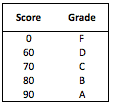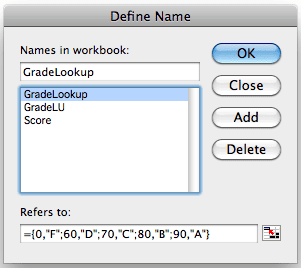There are several ways to turn student scores into letter grades. I recently came across a nested IF formula that did the trick, but it seemed rather complicated.

A better solution would be to use a VLOOKUP formula with a Grade Lookup Table, but then it occurred to me that the VLOOKUP formula could stand alone by using an Array Constant.

For all these solutions I’m using the following grade scale:

• 0 – 59 = F
• 60 – 69 = D
• 70 – 79 = C
• 80 – 89 = B
• 90 – 100 = A

## The Nested IF Solution

Using the scale above as a guide, the following nested IF formula will turn a score from 0 to 100 into the correct letter grade.

=IF(Score>=90,”A”,IF(Score>=80,”B”,IF(Score>=70,”C”,IF(Score>=60,”D”,”F”))))

This formula uses Score, which is a named range that contains all the student scores.

## A VLOOKUP with a Grade Lookup Table

The following formula will also give the correct letter grades.This formula has four arguments. Score refers to the student score that’s being looked up.

GradeLU is the Grade Lookup Table that’s on another worksheet and is a named range.

The two (2) means that a number from the second column will be returned from GradeLU.

The TRUE means the student score will be approximately matched to the first column from GradeLU. This is what allows a score of 72 to be matched to 70 and consequently return a letter grade of C.

## A VLOOKUP with an Array Constant

The Grade Lookup Table can be replaced with an array constant. In the formula above, the second argument, GradeLU, could be replaced with the following:

{0,”F”;60,”D”;70,”C”;80,”B”;90,”A”}

However, instead of typing this into the formula each time, we can create a Named Constant in the Define Name dialog box shown below.By creating GradeLookup as a named constant array, the formula can be shortened to:

This formula does not need to reference a separate Grade Lookup Table on a worksheet because all the values are located in the named array constant, GradeLookup, which is now located in Excel’s internal memory.

### Create a Named Constant Array

Bring up the Define Name dialog box and type in the name GradeLookup. Then delete the contents in the Refers to: text box and type in {0,”F”;60,”D”;70,”C”;80,”B”;90,”A”} exactly and click OK. Excel will add the equals sign (=).

How you access the Define Name dialog box depends on the version of Excel your using:

• In Excel 2003, and Excel 2008 and 2011 for Mac, use the menu selection Insert-Name-Define…
• In Excel 2007 and 2010 click the Ribbon tab Formulas and select Define Name.## 8 thoughts on “Grade Formulas in Excel”

1.Eric

Why not use

=CHOOSE(1+INT(Score/10),”F”,”F”,”F”,”F”,”F”,”F”,”D”,”C”,”B”,”A”,”A”)

But the LOOKUP works good if you grade on a curve.

1.Gregory

I thought about using CHOOSE, but was worried about the values from 1 to 10. You’ve solved that problem by adding a one (1) to INT(Score/10) so thanks for that.

2.Kevin Law

I get an value error message when attempting to employ GradeLookup as a constant named array. What am I doing wrong? More details might help.

1.Gregory

I’ll send you my worksheet. That should give you more detail.

3.RP

I have the same problem as Kevin when I try to use GradeLookup as a constant. I defined the constant array as stated and set up the VLookup as described, but I get the “#Value!” error. The formula seems to treat the constant array as one complete term, I think, since when I check the name it has inserted not only the “=” sign but also quotation marks around the array.

Would you mind describing how to use a constant (named) array in a little more detail than given above, since it seems like this may be a relatively common problem for people who are trying to replicate your method? I am sure it would be greatly appreciated since this is a very useful tip!

Thanks!
RP

1.Gregory

You probably have a slight, yet imperceptible error in the constant formula, which is very easy to do. I’ve updated the post to add the working Excel file that I referenced when I wrote the post. You can download my file and compare it to what you’ve done to see the difference.